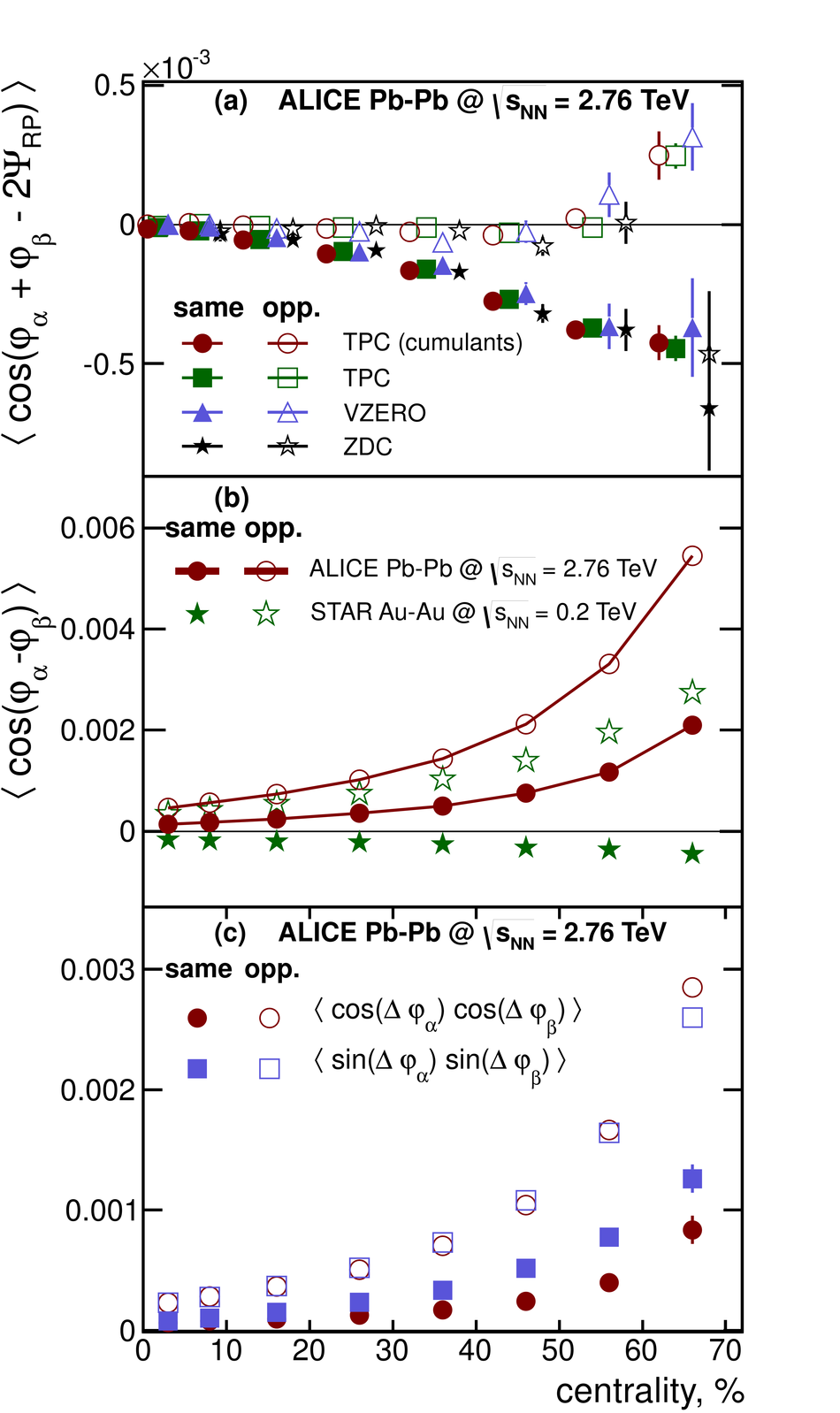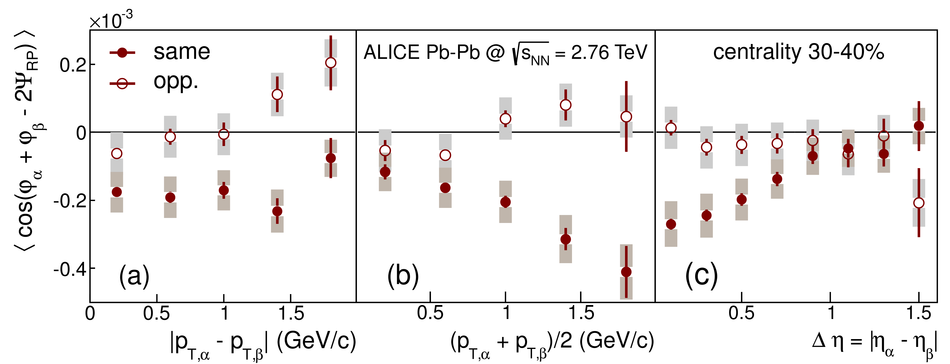# Charge separation relative to the reaction plane in Pb-Pb collisions at $\sqrt{s_{\rm NN}}= 2.76$ TeV

Measurements of charge dependent azimuthal correlations with the ALICE detector at the LHC are reported for Pb-Pb collisions at $\sqrt{s_{\rm NN}} = 2.76$ TeV. Two- and three-particle charge-dependent azimuthal correlations in the pseudo-rapidity range $|\eta| <~ 0.8$ are presented as a function of the collision centrality, particle separation in pseudo-rapidity, and transverse momentum. A clear signal compatible with a charge-dependent separation relative to the reaction plane is observed, which shows little or no collision energy dependence when compared to measurements at RHIC energies. This provides a new insight for understanding the nature of the charge dependent azimuthal correlations observed at RHIC and LHC energies.

Figures

## Figure 1

 (a) Centrality dependence of the correlator defined in Eq.2 measured with the cumulant method, and from correlations with the reaction plane estimated using the $\rm{TPC}$, the $\rm{ZDC}$ and the $\rm{VZERO}$ detectors. Only statistical errors are shown. The points are displaced slightly in the horizontal direction for visibility. (b) Centrality dependence of the two-particle correlator defined in Eq.3 compared to the STAR data. The width of the solid red lines indicates the systematic uncertainty of the ALICE measurement. (c) Decomposition of the correlators into $\langle{\cos\Dphi_a, \cos\Dphi_b}\rangle$ and $\langle{\sin\Dphi_a,\sin\Dphi_b}\rangle$ terms. The ALICE results in (b) and (c) are obtained with the cumulant method.## Figure 2

 The centrality dependence of the three-particle correlator defined in Eq.2. The circles indicate the ALICE results obtained from the cumulant analysis. The stars show the STAR data from . The triangles represent the genuine three-particle correlations ($\langle \cos(\varphi_{\alpha} + \varphi_{\beta} -2\varphi_c) \rangle$) from HIJING corrected for the experimentally measured v$_2\{2\}$. Points are displaced horizontally for visibility. A model prediction for the same sign correlations incorporating the Chiral Magnetic Effect for LHC energies is shown by the solid line. The shaded band represents the centrality dependence of the charge independent correlations.## Figure 3

 The three--particle correlator defined in Eq.2 as a function of (a) the transverse momentum difference, $|p_{{\rm t},\alpha}-p_{{\rm t},\beta}|$, (b)the average transverse momentum, $(p_{{\rm t},\alpha}+p_{{\rm t},\beta})/2$, and (c) the rapidity separation, $|\eta_{\alpha}-\eta_{\beta}|$, of the charged particle pair of same (closed symbols)and opposite (open symbols) sign.